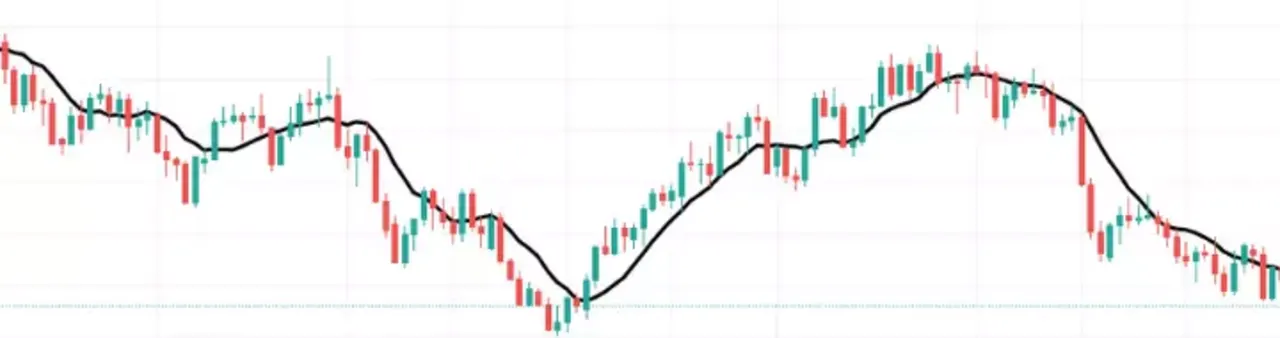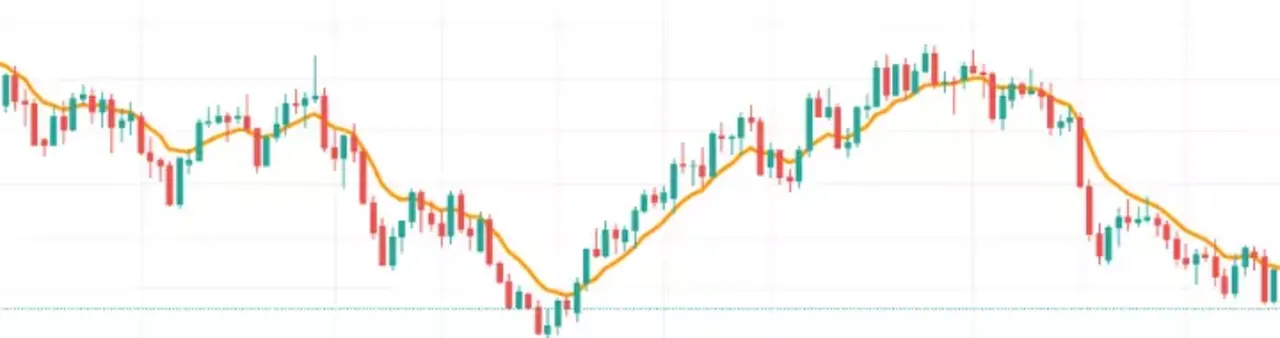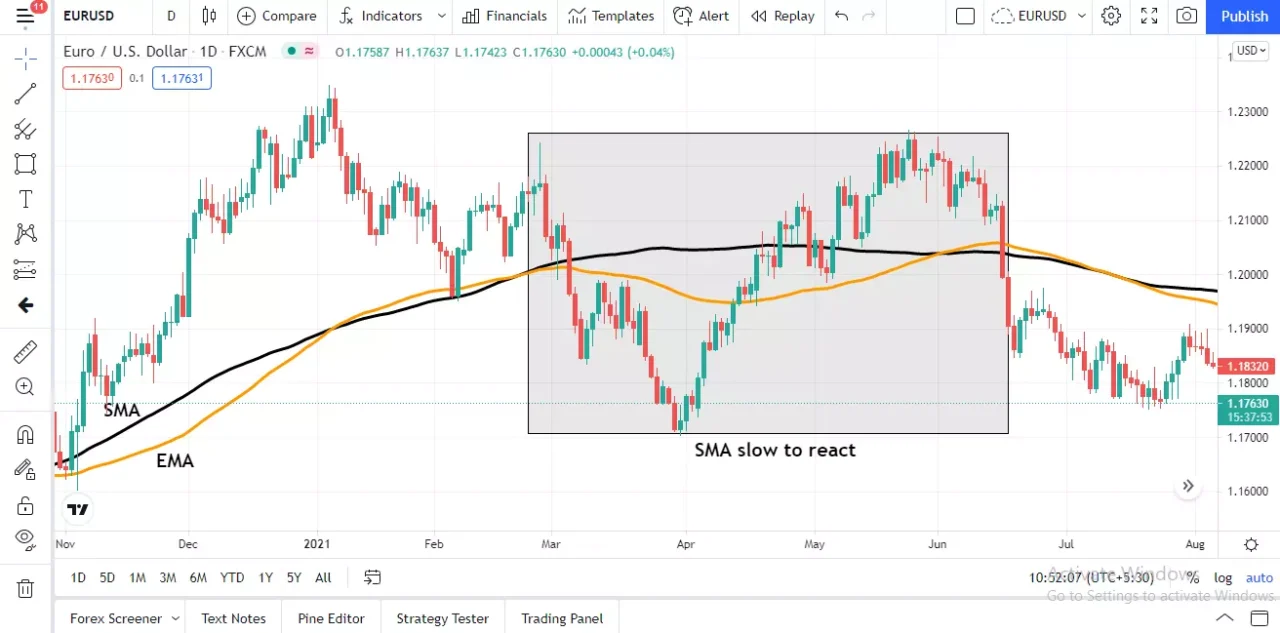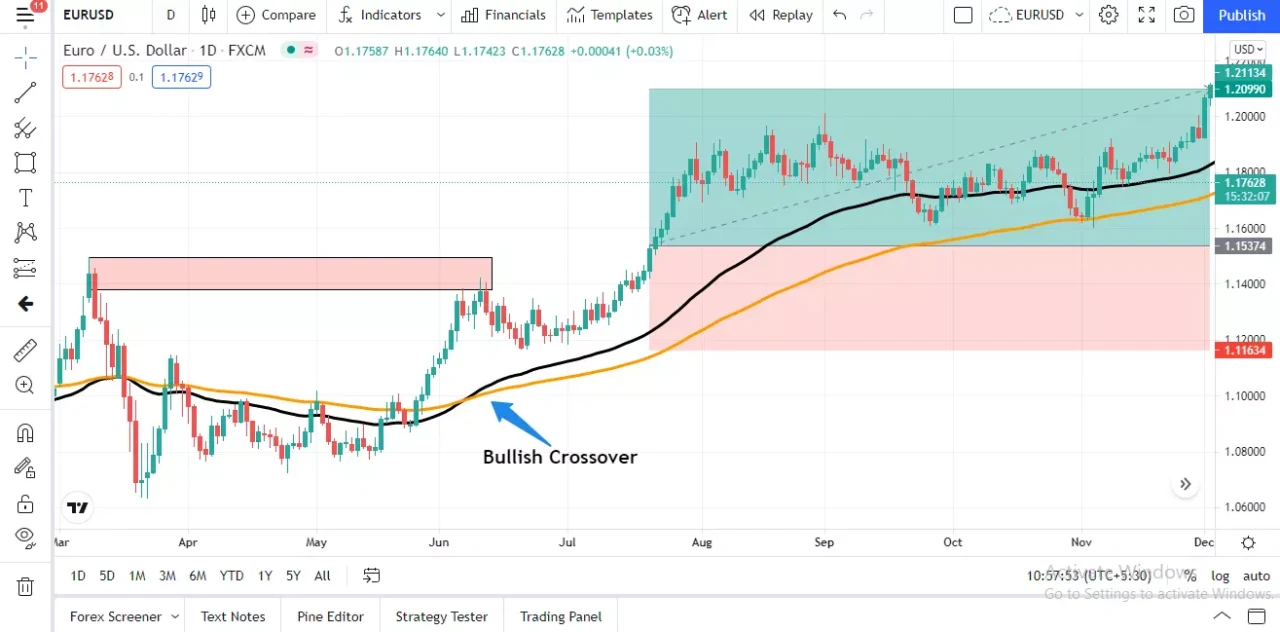# SMA vs EMA

Daksh Murkute | | |

Simple Moving Averages or Exponential Moving Average, which is better? This is one question that every trader asks at some point in their trading journey.

What if I told you that there is a moving average that will be way superior in helping you read price better, get sniper entries, and profit big just like I do? Sounds great, doesn’t it? If yes, then this blog post is just for you.

In this blog post, I am going to tell you everything you need to know about moving averages and which moving average between SMA and EMA is the better one.

If you want to learn how I use the moving average to capitalize on the price swings and make decent profits, then read this blog post till the end do not skip any part.

### Contents

What are moving averages?

What are SMAs and EMAs?

Calculating SMA and EMA

Which is better?

How you can use EMAs

Which one do you use?

#### Key Takeaways

###### iii. Both can be used to trade along with trend, pullbacks, and reversals.

Moving averages give us an average of the price over a period of time and the SMAs and the EMAs, are the most commonly used moving averages. There are some differences between the two and both moving averages shouldn't be used for the same purpose.

## What are moving averages?As the name suggests, moving averages give us the average of the prices of the financial assets over a period of time.

If these averages were to be plotted on a price chart and if each point were to be connected through a smoothened line, then we get a continuous sloping line known as a moving average.

Moving averages indicate all kinds of market phases, trending phase, and ranging phase and even helps spot reversals, depending upon how traders use this tool.

Moving averages are one of the most, if not the most, widely used technical indicators, and traders from around the globe use this to take a trade bias and make profits.

There are several types of moving averages but the most widely used moving averages are the Simple Moving Averages (SMA) and the Exponential Moving Averages (EMA).

## What are SMAs and EMAs?

SMAs and EMAs, as I mentioned above, are two types of moving averages and the main difference between the two lies in their calculation and the way it treats past price data.

To make the difference even more clear, let’s consider an illustration.

### 1. Simple Moving AverageHow will you decide whether a football player played well in a particular football match?

What you will do here is that you fetch the player’s past performance data, analyze it and then compare the latest performance with past data.

How will you analyze past performance? You can add up all the goals he scored and then divide it by the number of matches he played. This will give you an average of the number of goals the player scores in every match he plays.

This is exactly what the Simple Moving Average does. The SMA calculates the average of the prices within a particular time period. The average is calculated by adding the prices and dividing them by the number of days.

### 2. Exponential Moving AverageIf we are trying to figure out the performance of a player, will past performance be the only benchmark? What if the player has been in great form recently, shouldn’t that be given credit?

This is exactly what the Exponential Moving Average does.

The EMA also calculates an average of the prices over a period of time, but the calculation is not as simple as the SMA.

In the EMAs, recent price data is given additional weightage and this is what sets EMA apart from SMA.

Read ahead to understand how the SMAs and EMAs are calculated and what’s the technical difference between the two moving averages.

## Calculating SMA and EMAI hope that by now you have a general idea about how the two, SMAs and EMAs, are different from each other. But for the sake of better understanding, I will also get to the calculation part of the two moving averages.

### 1. How is SMA calculated?

SMA = Sum of all price data / No. of data points

To put it in simpler terms, if you want an SMA of say 5 days, then it adds the price data of all the 5 days, and this sum is divided by 5 i.e. the number of data points.

You can choose the length of SMAs according to your requirements and it can be on any timeframe.

If you see, the calculation for SMAs is the same as calculating a normal average, hence the name Simple Moving Average.

### 2. How is EMA calculated?

EMA = Price x k + Yesterday’s EMA x (1-k)

where k = 2/(Time Period+1)

Here, k is known as a weighted multiplier and this is what makes the difference between the two moving averages.

The calculations might seem complicated but you don’t have to actually sit and calculate EMAs every time. Luckily, the charting platform does all of this for us.

The length of EMAs too can be set according to our preferences and can be applied across all timeframes.

## Which is better?

If you asked me this question, then to me personally the EMAs are better and it is the only moving average that I always use in trading. I’ll tell you why.

There are basically two problems associated with SMAs and it is exactly where the EMAs have the edge.

### 1. Weightage given to price data

Let’s consider a currency pair and 10 days of its price data - 150, 50, 40, 30, 50, 40, 20, 50, 70, and 60. The average of this will be 56.

Now suppose, we have to add the price for the 11th day, so the first price data in this set will be removed. The new set will be - 50, 40, 30, 50, 40, 20, 50, 70, 60, and 50. The new average will be 46.

Do you notice the drastic decrease? This change happened just because one price data was removed and another one was added. This is the problem with SMAs, it gives equal weightage to all price data in the set.

The EMAs on the other hand give additional weightage to the most recent price data. If you consider a 10 day EMA, then you will notice that the EMA gives 18% weightage to the most recent price data whereas in a 10 day SMA it would get just 10% weightage.

The EMA gives more weightage to the most recent price data and it reduces the weightage given to older price data, but the SMAs do not do this and each price data gets equal weightage.

This is the reason why we see such drastic changes in the SMA values as and when newer price data is added to the set to calculate SMAs.

The transition from old to new in the EMAs is smoother as older price data with lesser weightage are discarded, hence having no significant changes.

It is because of this we notice whipsaws by SMAs and this, in turn, makes things difficult for us traders as we are stuck in the middle of nowhere trying to understand what exactly the price is doing.### 2. Reaction to price movements

Which moving average will be faster to react to the price movements? Of course the EMAs. You might ask what difference this will make, let me tell you that it makes a big difference.

Consider that the price of any particular currency pair or any financial asset is in a range and the price doesn’t close much far away from the previous close.

What if one sudden day there is a big move to the upside and the price closes way higher. Both moving averages will react accordingly, but the EMAs will be quicker to absorb this wide move over the SMA.

It will take some time before the SMAs actually show some movement and will move in the direction of the trend. This is why it is said that SMAs are lagging.

In today’s times of trading, speed is what makes the difference. Everyone has access to information and the markets but what sets winners apart is who acts on it faster.

There are going to be innumerable traders around the globe using moving averages to trade, so won’t it be better if you used a moving average that reacts faster to the price movements?

## How you can use EMAsNow that we have discussed moving averages in detail and have seen how EMAs are better than SMAs, let’s get to the practical side of EMAs and how you can use this to take trades.

The first and foremost usage of EMAs is for trend identification. If the price is above the EMA then it is bullish and if it is below it then it is bearish.

It should be noted that this alone isn’t enough to take trades. You have to look at certain other things also before you actually pull the trigger.

Next is reversals. EMAs will help you identify reversals in two ways, price and EMA crossover or EMA crossovers of either 2 or 3 EMAs.

The advantage EMAs will have SMAs in reversals is that since it reacts faster towards the price movements, traders using this can spot reversals faster.

You can also get better entries if you trade pullbacks with EMAs.

The advantage that the EMAs have over the SMAs is because EMAs react to price quicker. Hence, everything that the SMA lacks, is what you will find in EMAs.

## Which one do you use?

Do let me know which type of moving you use and also mention the reason for the same.

Share this blog post with other fellow traders and let them also understand why EMAs and better than SMAs.

Feel free to reach out to me through the comments section for any questions, queries, or anything in general.